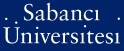# "Equivalent" material properties for designing ionic polymer metal composite actuators by equivalent bimorph beam theory

Çilingir, Halime Didem (2008) "Equivalent" material properties for designing ionic polymer metal composite actuators by equivalent bimorph beam theory. [Thesis]PDF - Registered users only - Requires a PDF viewer such as GSview, Xpdf or Adobe Acrobat Reader4Mb

## Abstract

This thesis addresses the Ionic Polymer Metal Composite (IPMC) actuators and two “equivalent” materials parameters for their design and performance assessments: electromechanical coupling coefficient and elastic modulus. The “equivalent” parameters not being material constants are derived from equivalent bimorph beam model. The Nafion membrane based IPMC actuator strips of several thicknesses are manufactured by electrochemical platinization method. The effect of the thickness and operating voltage on the equivalent coupling coefficient is demonstrated by using a design of experiment of three and five levels of the two factors, respectively. Experiments and finite element analyses using MD.NASTRAN are used to evaluate the tip displacement and the coupling coefficient for which response surface (RS) approximation as function of the thickness and voltage are constructed. Experiments and predictions indicate that thickness and voltage are interacting major factors for maximum tip displacement. The equivalent coupling coefficient is primarily driven by the thickness, and the voltage appears to contribute as the thickness increases. Initial curvature of the strips before excitation is also shown to be a factor for “equivalent” coupling coefficient, it is not, however sufficient to explain the variation in the experimental data. Correction factor approach is proposed and applied to the straight beam tip displacement RS that filters out experimental variation. Corrected RS enables to include the pre-imposed initial curvature as design parameter along with the actuator thickness and the operating peak voltage when predicting the tip displacement and the equivalent coupling coefficient. IPMC actuator “equivalent” elastic modulus is also determined by using blocking force data. The “equivalent” properties, electromechanical coefficient and young’s modulus by the equivalent bimorph beam model works reasonably well in calculating the actuation force at the tip by MD.NASTRAN. These “equivalent” material properties can be easily implemented in preliminary design of actuator made of IPMC.

Item Type: Thesis IPMC. -- Equivalent bimorph beam model. -- Nastran. -- Finite element analysis. -- İPMK. -- Eşdeğer bimorf kiriş modeli. -- Nastran. -- Sonlu elemanlar analizi. T Technology > TA Engineering (General). Civil engineering (General) > TA401-492 Materials of engineering and construction. Mechanics of materials 14042 IC-Cataloging 15 Jun 2010 16:15 17 Aug 2012 11:52

Repository Staff Only: item control page